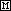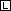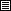Preparing Solutions

## Preparing Solutions

Solutions are commonly made up in the laboratory from solid materials, from liquids or from other solutions. The descriptions below assume knowledge of the calculations required to determine solution concentrations, the ability to accurately weigh solids and to pipet liquids.

From solid material
 (1) Determine the concentration and amount of solution required for the experiment. (2) Calculate the amount of solute required to prepare the desired solution. (3) Weigh out the amount of solute calculated in step (2) and obtain a volumetric flask of appropriate volume. (4) Add the solute to the volumetric flask. (5) Fill the volumetric flask approximately two thirds full, stopper and mix. Do this by inverting the flask, shaking and returning the flask to upright. Do this ten times. Make sure to hold the stopper in the flask. (6) Carefully fill the flask to the mark etched on the neck of the flask. Use a wash bottle or medicine dropper if necessary. (7) Mix the solution thoroughly by stoppering the flask securely and inverting it ten to twelve times.

From a liquid or other solution
 (1) Determine the concentration and amount of solution required for the experiment. (2) Calculate the amount of stock solution or liquid required to prepare the desired solution (a stock solution is one with a known concentration greater than the solution you are preparing). (3) Use a pipet to measure the amount of solution or liquid calculated in step (2). (4) Add the solution or liquid to a volumetric flask of appropriate volume. (5) Fill the volumetric flask approximately two thirds full and mix. (6) Carefully fill the flask to the mark etched on the neck of the flask. Use a wash bottle or medicine dropper if necessary. (7) Mix the solution thoroughly by stoppering the flask securely and inverting it ten to twelve times.